Next: Non-isolated systems Up: Newtonian mechanics Previous: Newton's second law of

# Newton's third law of motion

Consider a system of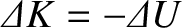mutually interacting point objects. Let the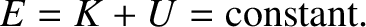th object, whose mass is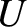, be located at position vector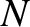. Suppose that this object exerts a force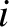on the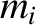th object. Likewise, suppose that theth object exerts a force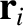on theth object. Newton's third law of motion essentially states that these two forces are equal and opposite, irrespective of their nature. In other words,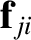(2.23)

(See Figure 2.2.) One corollary of Newton's third law is that an object cannot exert a force on itself. Another corollary is that all forces in the universe have corresponding reactions. The only exceptions to this rule are the fictitious forces that arise in non-inertial reference frames (e.g., the centrifugal and Coriolis forces that appear in rotating reference frames--see Chapter 6). Fictitious forces do not generally possess reactions.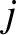Newton's third law implies action at a distance. In other words, if the force that objectexerts on objectsuddenly changes then Newton's third law demands that there must be an immediate change in the force that objectexerts on object. Moreover, this must be true irrespective of the distance between the two objects. However, we now know that Einstein's special theory of relativity forbids information from traveling through the universe faster than the velocity of light in vacuum (Rindler 1977). Hence, action at a distance is also forbidden. In other words, if the force that objectexerts on objectsuddenly changes then there must be a time delay, which is at least as long as it takes a light ray to propagate between the two objects, before the force that objectexerts on objectcan respond. Of course, this means that Newton's third law is not, strictly speaking, correct. However, as long as we restrict our investigations to the motions of dynamical systems over timescales that are long compared to the time required for light rays to traverse these systems, Newton's third law can be regarded as being approximately correct.

In an inertial frame, Newton's second law of motion applied to theth object yields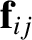(2.24)

Note that the summation on the right-hand side of this equation excludes the case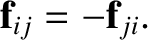, because theth object cannot exert a force on itself. Let us now take this equation and sum it over all objects. We obtain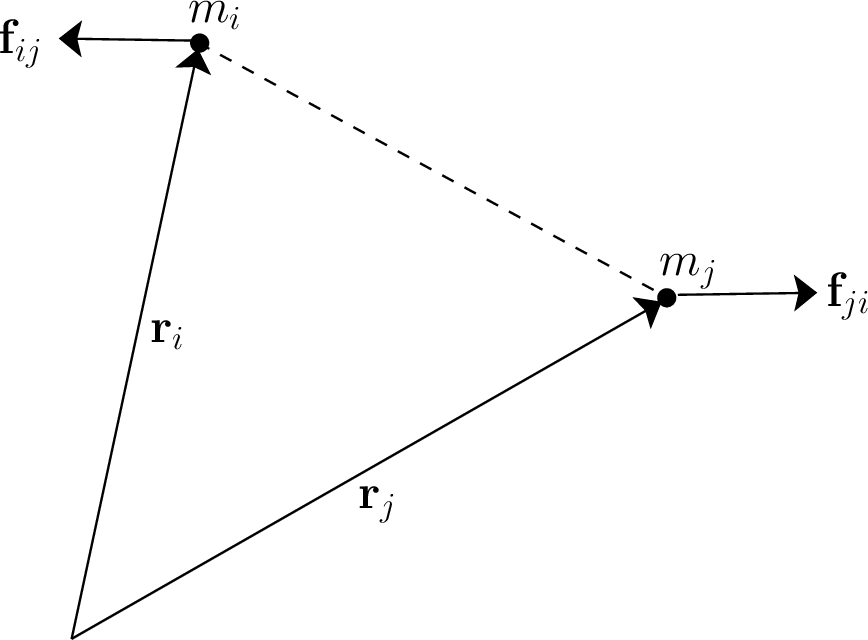(2.25)

Consider the sum over forces on the right-hand side of the preceding equation. Each element of this sum--, say--can be paired with another element--, in this case--which is equal and opposite, according to Newton's third law. In other words, the elements of the sum all cancel out in pairs. Thus, the net value of the sum is zero. It follows that Equation (2.25) can be written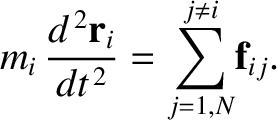(2.26)

where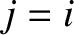is the total mass. The quantity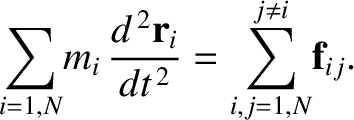is the vector displacement of the center of mass of the system, which is an imaginary point whose coordinates are the mass-weighted averages of the coordinates of the objects that constitute the system: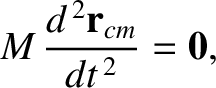(2.27)

According to Equation (2.26), the center of mass of the system moves uniformly in a straight line, in accordance with Newton's first law of motion, irrespective of the nature of the forces acting between the various components of the system.

Now, if the center of mass moves uniformly in a straight line then the center of mass velocity,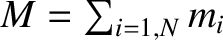(2.28)

is a constant of the motion. However, the momentum of theth object takes the form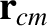. Hence, the total momentum of the system is written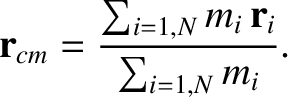(2.29)

A comparison of Equations (2.28) and (2.29) suggests that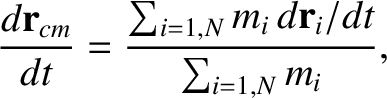is also a constant of the motion. In other words, the total momentum of the system is a conserved quantity, irrespective of the nature of the forces acting between the various components of the system. This result (which only holds if there is zero net external force acting on the system) is a direct consequence of Newton's third law of motion.

Taking the vector product of Equation (2.24) with the position vector, we obtain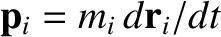(2.30)

The right-hand side of this equation is the net torque about the origin that acts on objectas a result of the forces exerted on it by the other objects. It is easily seen that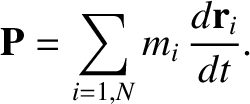(2.31)

where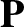(2.32)

is the angular momentum of theth object about the origin of our coordinate system. Moreover, the total angular momentum of the system (about the origin) takes the form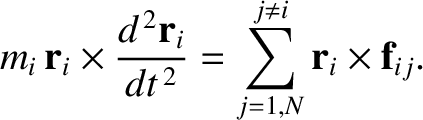(2.33)

Hence, summing Equation (2.30) over all particles, we obtain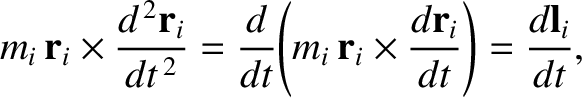(2.34)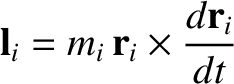Consider the sum on the right-hand side of Equation (2.34). A general term,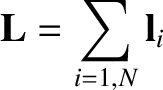, in this sum can always be paired with a matching term,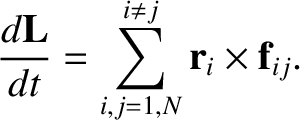, in which the indices have been swapped. Making use of Equation (2.23), we can write the sum of a general matched pair as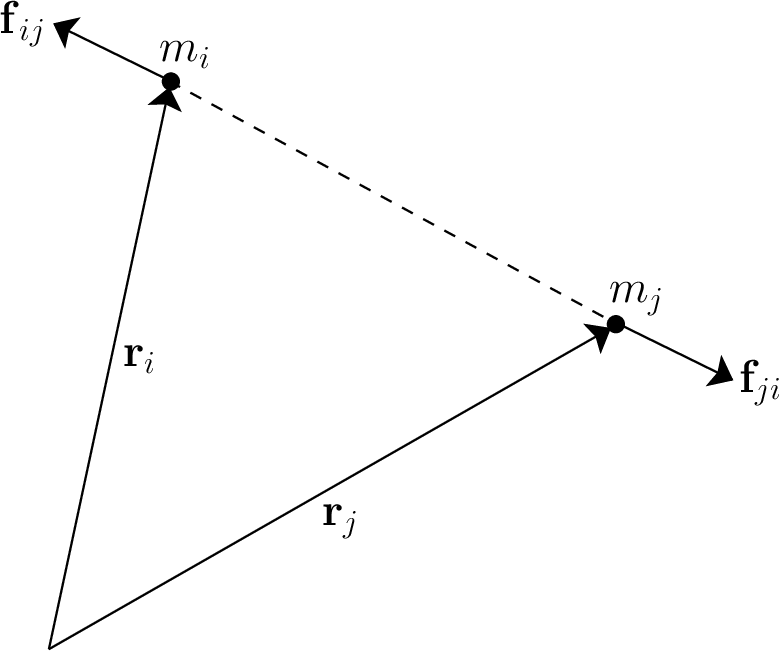(2.35)

Let us assume that the forces acting between the various components of the system are central in nature, so thatis parallel to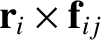. In other words, the force exerted on objectby objecteither points directly toward, or directly away from, object, and vice versa. (See Figure 2.3.) This is a reasonable assumption, because virtually all of the forces that we encounter in celestial mechanics are of this type (e.g., gravity). It follows that if the forces are central then the vector product on the right-hand side of the previous expression is zero. We conclude that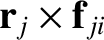(2.36)

for all values ofand. Thus, the sum on the right-hand side of Equation (2.34) is zero for any kind of central force. We are left with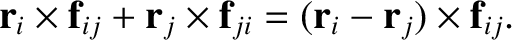(2.37)

In other words, the total angular momentum of the system is a conserved quantity, provided that the different components of the system interact via central forces (and there is zero net external torque acting on the system).Next: Non-isolated systems Up: Newtonian mechanics Previous: Newton's second law of
Richard Fitzpatrick 2016-03-31